KUULCHAT
SCIENCE MOCK

#### OBJECTIVE TEST

1.

Which of the following statements about a second class lever is correct? The

A.

pivot is between the load and the effort.

B.

pivot and the load are at the same position.

C.

load is between the effort and the pivot.

D.

effort is between the load and the pivot.

2.

17. A stone displaced 20cm3 of water when immersed in the water. If the mass of the stone is 40g. find the density of the stone

A.

60gcm-3

B.

40gcm-3

C.

2gcm-3

D.

0.5g cm-3

3.

An example of a body that does not produce its own light is the

A.

moon.

B.

sun.

C.

star.

D.

fire fly.

4.

In electronic circuits, LEDs are used to indicate the absence or presence of

A.

voltage source.

B.

p-n junction.

C.

electric current.

D.

emitted and collector.

5.

The farming system that makes effective use of available soil nutrients in a growing season is

A.

crop rotation.

B.

land rotation.

C.

mixed cropping.

D.

mixed farming.

6.

A structure in the human reproductive system which stores sperms temporarily is the

A.

epididymis.

B.

penis.

C.

scrotum.

D.

vulva.

7.

The basic unit of life is the

A.

cell.

B.

nucleus.

C.

organ.

D.

tissue.

8.

The gas produced when glucose is oxidized during aerobic respiration is

A.

Hydrogen

B.

Nitrogen

C.

Oxygen

D.

Carbon dioxide

9.

A fruit in which the pappus has been modified into a parachute structure is dispersed by

A.

explosion

B.

mammals

C.

water

D.

wind

10.

Which of the following statements about aerobic respiration is correct?

A.

The by-product are carbon dioxide and water.

B.

Little amount of energy is produced.

C.

Oxygen is not required.

D.

The by-products are alcohol and carbon dioxide.

11.

The feeling of soil between fingers is used to determine the

A.

texture of the soil.

B.

drainage of the soil.

C.

capillary of the soil.

D.

water holding capacity of the soil.

12.

Which of the following gases supports combustion?

A.

Hydrogen

B.

Oxygen

C.

Carbon dioxide

D.

Carbon monoxide

13.

Movements of the ribs is brought about by the

A.

pleural cavity.

B.

intercostal muscle.

C.

larynx.

D.

trachea.

14.

Which of the following devices is made of semiconductor?

A.

Capacitor

B.

Inductor

C.

Resistor

D.

Transistor

15.

The systematic name of NO2 is

A.

nitrogen oxide.

B.

nitrogen (i) oxide.

C.

nitrogen (ii) oxide.

D.

nitrogen (iv) oxide.

16.

Which of the following soil type has the highest capillarity?

A.

Clay

B.

Loam

C.

Sand

D.

Silt

17.

The elements in the periodic table are arranged according to their

A.

atomic number

B.

atomic mass

C.

mass number

D.

neutron number

18.

The ovules in a flower develop to form the

A.

fruit.

B.

leaf.

C.

seed.

D.

stem.

19.

How many atoms are present in CaCl2?

A.

2

B.

3

C.

4

D.

5

20.

An example of a plant micro-nutrient is

A.

calcium.

B.

copper.

C.

magnesium.

D.

potassium.

21.

A rheostat is used in an electric circuit in order to

A.

increase electric current only.

B.

decrease electric current only.

C.

keep electric current constant.

D.

increase and decrease electric current.

22.

One of the characteristics of wind pollinated flowers is that they

A.

are large and conspicuous.

B.

are usually scented.

C.

have nectar.

D.

have feathery stigma.

23.

Which of the following source(s) of energy is/are renewable?

I. Solar

II. Crude oil

III. Wind

A.

I only

B.

I and II only

C.

I and III ONLY

D.

I, II and III

24.

The housefly is an agent for the spread of

A.

cholera.

B.

malaria.

C.

measles.

D.

tuberculosis.

25.

The second large in the life cycle of a mosquito is the

A.

egg.

B.

imago.

C.

larva.

D.

pupa.

26.

The part of a living cell which is semi-permeable is

A.

cytoplasm.

B.

nucleus.

C.

protoplasm.

D.

cell membrane.

27.

A disease-causing organism that is most difficult to control in crop production is

A.

bacterium

B.

fungus

C.

nematode

D.

virus

28.

The type of soil which become water-logged after rainfall is made up of a large amount of

A.

silt.

B.

sand.

C.

gravel.

D.

clay.

29.

The function of the hair in the nose of humans is to

A.

make breathing easier.

B.

keep nose moist.

C.

filter dust from the air breathed in.

D.

keep the nose warm.

30.

Sperms produced by the testes in humans are temporarily stored in the

A.

epididymis.

B.

prostate gland.

C.

seminal vesicles.

D.

sperm duct.

31.

Which of the following particles constitute matter?

I. Atoms

II. Molecules

III. Ions

A.

I only

B.

II only

C.

II and III only

D.

I, II and III

32.

The organelle which occupies the largest portion of a plant cell is the

A.

chloroplast.

B.

mitochondrion.

C.

nucleus.

D.

vacuole.

33.

Which of the following statements about the properties of water are correct? It

I. is colourless and tasteless

II. is neutral to litmus paper

III. turns red litmus paper blue

IV. is a universal solvent

A.

I and II only

B.

I and III only

C.

I, II and IV only

D.

I, III and IV only

34.

Which of the following type of teeth is used by mammals to tear food material?

A.

Canines

B.

Incisors

C.

Molars

D.

Premolars

35.

The release of a mature egg from an ovary into the fallopian tube in humans is called

A.

copulation.

B.

ejaculation.

C.

menstruation.

D.

ovulation.

36.

The process by which metabolic wastes are removed from the body is called

A.

respiration

B.

excretion

C.
ingestion

D.

egestion

37.

Solid non-metals normally break into pieces when hammered because they are

A.

brittle

B.

ductile

C.

lustrous

D.

malleable

38.

The physical arrangement of soil particles into aggregate is termed

A.

soil porosity

B.

soil profile

C.

soil structure

D.

soil texture

39.

When a green leaf is placed in a test tube containing ethanol and heated over a water bath the leaf

A.

becomes soft

B.

changes colour to brown

C.

changes colour to blue-black

D.

is decolorized

40.

Which of the following elements is a macro-nutrient?

A.

Copper

B.

Manganese

C.

Zinc

D.

Sulphur

#### THEORY QUESTIONS

1.

(a) (i) State two physical properties of water.

(ii) Using litmus paper, explain why water is neutral.

(b) Give one function of each of the following nutrients in plants:

(i) Nitrogen;

(ii) Potassium.

(c) (i) State two human activities that disrupt of the carbon cycle.

(ii) State two effects of the disruption of the carbon cycle on the environment.

(d) (i) What is density of a body?

(ii) A body of mass 50kg has a density of 2kg/m3. Calculate the volume of the body.

2.

(a) In an experiment the surfaces of three iron nails were cleaned dry and placed in three separate test tubes A, B and C as shown in the diagram.

Study the diagram carefully and answer the questions that follow.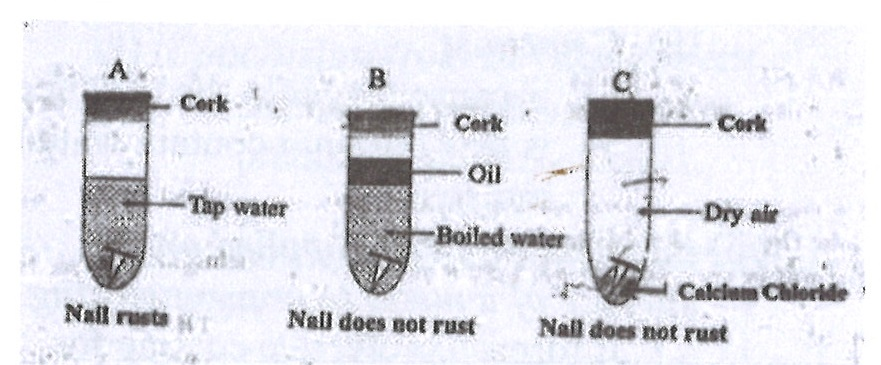After three days the nail in the test tube A was found to have rusted while the nail in test tubes B and C did not rust.

(i) Suggest an aim for the experiment.

(ii) Why was the water in test tube B boiled?

(iii) State the function of the oil on top of the water in test tube B.

(iv) What is the purpose of the calcium chloride in test tube C?

(v) Why did the nail in test tube A rust?

(vi) Why did the nail in test tube

(α) B not rusted?

(β) C not rusted?

(vii) From the experiment, explain why oil is applied on the surface of a metal to prevent rusting.

(b) In an experiment, the following activities were carried out on two green leaves A and B. Leaf A was taken from a plant placed in the sunlight for some time while leaf B was taken from a plant placed in a dark cupboard for 24 hours.

Activity

I. Leaves dipped in boiling water for 1 minute;

II. Leaves dipped in warm alcohol;

III. Leaves washed in cold water;

IV. Leaves dipped in iodine solution.

After dipping in the iodine solution, it was observed that leaf A changed colour.

Study the activities carefully and answer the questions that follow.

(i) Suggest an aim for the experiment.

(ii) Explain briefly why each of the following activities were carried out:

(α) I;

(β) II;

(γ) III.

(iii) State the colour change of leaf A.

(iv) Explain why leaf A changed colour?

(v) Why is it necessary to de-starch the plant before starting the experiment?

(vi) From the above experiment, what conclusion(s) can be drawn.

(c) You are provided with two dry soil samples A and B, a measuring cylinder, beakers, funnels, filter paper, beam balance, stop watch and water. With the aid of a labelled diagram, describe an experiment to find out which of the two soil samples can hold more water.

(d) The diagram below is an illustration of an experiment that was carried out. In the experiment three pins 1, 2 and 3 were fixed with candle wax onto a metal bar and one end of the bar was heated by means of boiling water. A, B and C are thermometers inserted in holes along the bar to measure the temperature at the various points.

Study the diagram carefully and answer the questions that follow.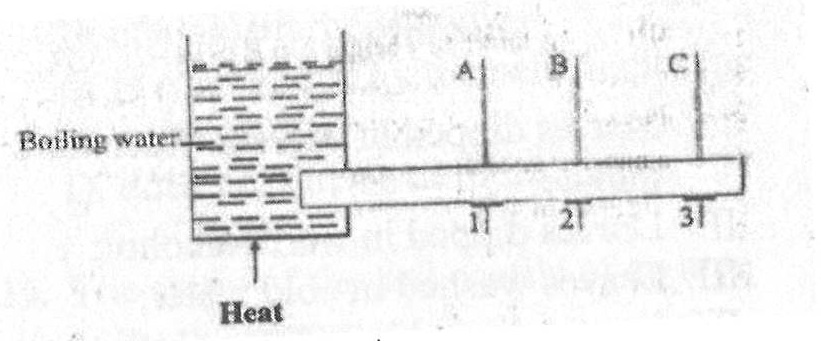(i) Suggest an aim for the experiment.

(ii) What is the temperature of the boiling water?

(iii) State the observation that would be made about the pins 1, 2 and 3.

(iv) State the observation that would be made about the temperatures recorded by the thermometers A, B and C.

(v) State how heat from the sun is transmitted to the earth.

3.

(a) Name four weather measuring instruments.

(b) Name the stages in the life cycle of a mosquito.

(c) (i) List two properties of water.

(ii) Explain why it is advisable to wash clothes with soft water.

(d) State three ways in which soil profile is important.

4.

(a) The diagram below is an illustration of a longitudinal section of a canine tooth in humans.

Study the diagram carefully and answer the questions that follow.(i) Name each of the parts labelled I, II, III and IV.

(ii) What is the function of each of the parts labelled I and III?

(iii) Which of the labelled parts could be affected by tooth decay?

(iv) State three ways by which tooth decay may be prevented.

(b) The diagram below is an illustration of a scientific phenomenom which occurs in nature.

Study the diagram carefully and answer the questions that follow.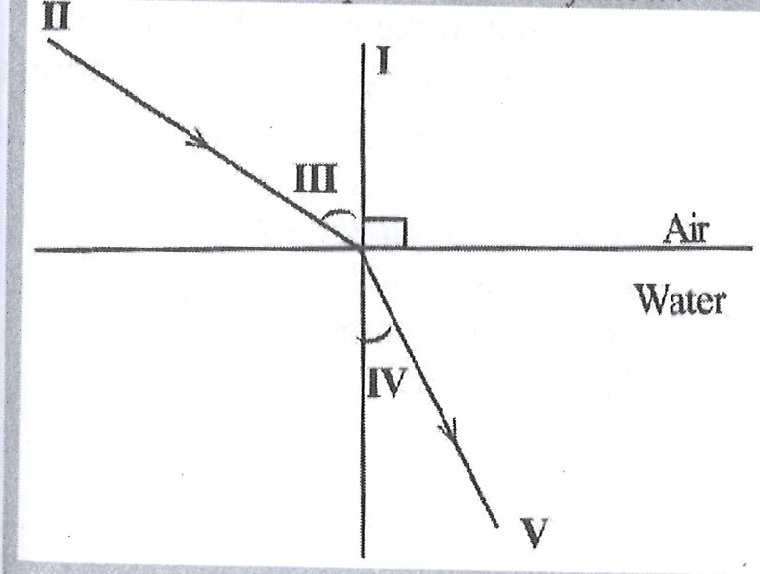(i) What phenomenom does the diagram illustrate?

(ii) Identify each of the parts labelled I, II, III, IV and V.

(iii) Explain why an object at the bottom of a pond appears closer to the surface than it actually is.

(c) The diagram below is an illustration of an experiment performed to separate the components of muddy water.

Study the diagram carefully and answer the questions that follow.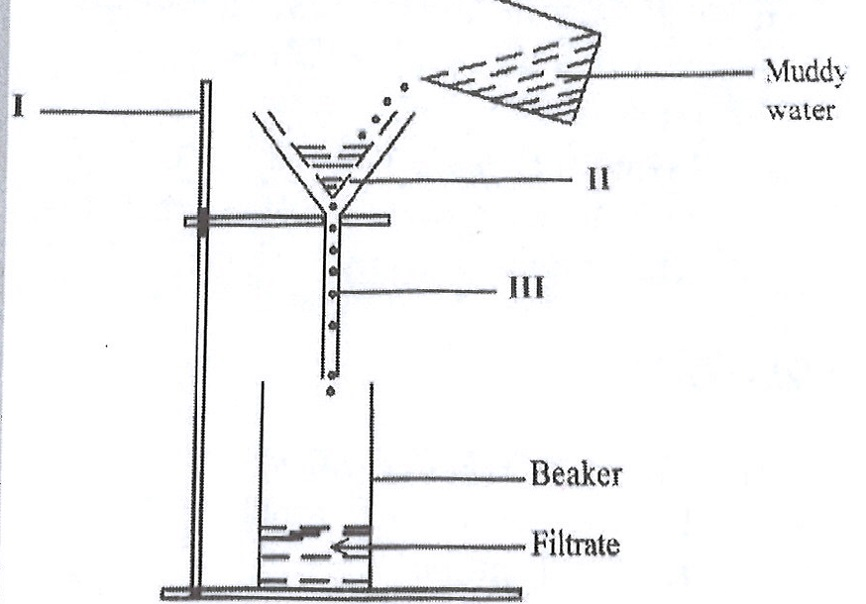(i) Name each of the parts labelled I, II, and III.

(ii) State the function of the part labelled II.

(iii) Name the substance obtained as the filtrate.

(iv) State three physical properties of the filtrate.

(v) Name two other materials that could be used in place of the part labelled II.

(d) The diagram below is an illustrates a farmland on a sloppy area.

Study the diagram carefully and answer the questions that follow.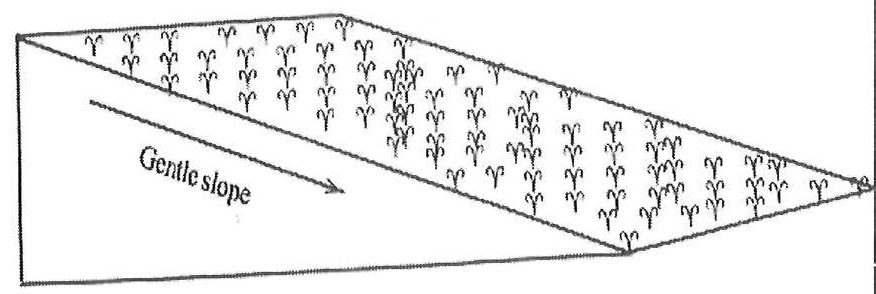(i) What process is likely to occur on the farmland when it rains heavily?

(ii) State two farming practices that can also lead to the process mentioned in (i).

(iii) List four farming practices that could be used to control the process mentioned in (i).

(iv) Mention three soil resources that would be depleted from the farmland when it rains heavily.

5.

(a) (i) What are derived quantities?

(ii) State the S.I units of the following quantities:

(α) area;

(β) volume.

(b) (i) State two factors necessary for photosynthesis.

(ii) Explain the functions of each of the factors stated in (i).

(c) Explain each of the following terms:

(i) Soft water;

(ii) Hard water.

(d) State three reasons why some seeds are nursed.

6.

(a) (i) What is a magnetic field?

(ii) Name two methods of making magnets.

(b) Explain briefly the term teenage pregnancy.

(c) Write the formula for each of the following compounds:

(i) calcium chloride;

(ii) copper (I) oxide;

(iii) nitrogen (IV) oxide;

(iv) ammonia.

(d) (i) List three physical properties of soil.

(ii) What is the texture of clayey soil?